MathematicsSolving BVP Problems

This section describes:

Example: Mathieu's Equation

This example determines the fourth eigenvalue of Mathieu's Equation. It illustrates how to write second-order differential equations as a system of two first-order ODEs and how to use `bvp4c` to determine an unknown parameter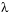.

The task is to compute the fourth (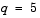) eigenvalueof Mathieu's equation

•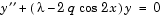Because the unknown parameteris present, this second-order differential equation is subject to three boundary conditions

•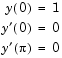Note    The demo `mat4bvp` contains the complete code for this example. The demo uses nested functions to place all functions required by `bvp4c` in a single M-file. To run this example type `mat4bvp` at the command line. See BVP Solver Basic Syntax for more information.

1. Rewrite the problem as a first-order system. To use `bvp4c`, you must rewrite the equations as an equivalent system of first-order differential equations. Using a substitution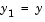and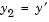, the differential equation is written as a system of two first-order equations
•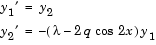1. Note that the differential equations depend on the unknown parameter. The boundary conditions become

•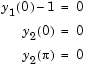1. Code the system of first-order ODEs. Once you represent the equation as a first-order system, you can code it as a function that `bvp4c` can use. Because there is an unknown parameter, the function must be of the form
• ```dydx = odefun(x,y,parameters)
```
1. The following code represents the system in the function, `mat4ode`. Variable `q` is shared with the outer function:

• ```function dydx = mat4ode(x,y,lambda)
dydx = [ y(2)
-(lambda - 2*q*cos(2*x))*y(1) ];
end   % End nested function mat4ode
```

See Finding Unknown Parameters for more information about using unknown parameters with `bvp4c`.

1. Code the boundary conditions function. You must also code the boundary conditions in a function. Because there is an unknown parameter, the function must be of the form
• ```res = bcfun(ya,yb,parameters)
```
1. The code below represents the boundary conditions in the function, `mat4bc`.

• ```function res = mat4bc(ya,yb,lambda)
res = [  ya(2)
yb(2)
ya(1)-1 ];
```
1. Create an initial guess. To form the guess structure `solinit` with `bvpinit`, you need to provide initial guesses for both the solution and the unknown parameter.
1. The function `mat4init` provides an initial guess for the solution. `mat4init` uses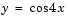because this function satisfies the boundary conditions and has the correct qualitative behavior (the correct number of sign changes).

• ```function yinit = mat4init(x)
yinit = [  cos(4*x)
-4*sin(4*x) ];
```

In the call to `bvpinit`, the third argument, `lambda`, provides an initial guess for the unknown parameter.

• ```lambda = 15;
solinit = bvpinit(linspace(0,pi,10),@mat4init,lambda);
```

This example uses `@` to pass `mat4init` as a function handle to `bvpinit`.

 Note    See the `function_handle` (`@`), `func2str`, and `str2func` reference pages, and the Function Handles chapter of "Programming and Data Types" in the MATLAB documentation for information about function handles.

1. Apply the BVP solver. The `mat4bvp` example calls `bvp4c` with the functions `mat4ode` and `mat4bc` and the structure `solinit` created with `bvpinit`.
• ```sol = bvp4c(@mat4ode,@mat4bc,solinit);
```
2. View the results. Complete the example by displaying the results:
1. Print the value of the unknown parameterfound by `bvp4c`.
• ```    fprintf('The fourth eigenvalue is approximately %7.3f.\n',...
sol.parameters)
```
2. Use `deval` to evaluate the numerical solution at 100 equally spaced points in the interval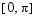, and plot its first component. This component approximates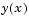.
• ```    xint = linspace(0,pi);
Sxint = deval(sol,xint);
plot(xint,Sxint(1,:))
axis([0 pi -1 1.1])
title('Eigenfunction of Mathieu''s equation.')
xlabel('x')
ylabel('solution y')
```
• See Evaluating the Solution at Specific Points for information about using `deval`.

The following plot shows the eigenfunction associated with the final eigenvalue= 17.097.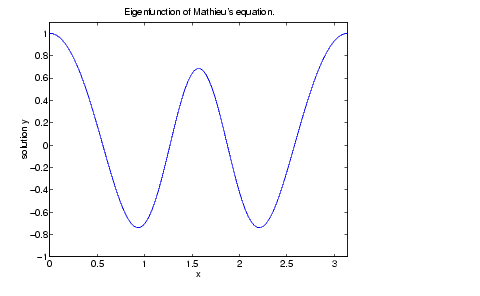Finding Unknown Parameters

The `bvp4c` solver can find unknown parameters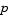for problems of the form

•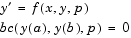You must provide `bvp4c` an initial guess for any unknown parameters in the vector `solinit.parameters`. When you call `bvpinit` to create the structure `solinit`, specify the initial guess as a vector in the additional argument `parameters`.

• ```solinit = bvpinit(x,v,parameters)
```

The `bvp4c` function arguments `odefun` and `bcfun` must each have a third argument.

• ```dydx = odefun(x,y,parameters)
res = bcfun(ya,yb,parameters)
```

While solving the differential equations, `bvp4c` adjusts the value of unknown parameters to satisfy the boundary conditions. The solver returns the final values of these unknown parameters in `sol.parameters`. See Example: Mathieu's Equation.

Evaluating the Solution at Specific Points

The collocation method implemented in `bvp4c` produces a C1-continuous solution over the whole interval of integration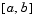. You can evaluate the approximate solution,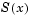, at any point inusing the helper function `deval` and the structure `sol` returned by `bvp4c`.

• ```Sxint = deval(sol,xint)
```

The `deval` function is vectorized. For a vector `xint`, the `i`th column of `Sxint` approximates the solution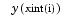.Boundary Value Problem Solver Using Continuation to Make a Good Initial Guess© 1994-2005 The MathWorks, Inc.